Search by Topic

Resources tagged with Generalising similar to Uncanny Triangles:

Filter by: Content type:
Age range:
Challenge level:

There are 141 results

Broad Topics > Using, Applying and Reasoning about Mathematics > GeneralisingTiling

Age 7 to 11 Challenge Level:

An investigation that gives you the opportunity to make and justify predictions.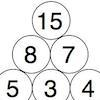Build it Up

Age 7 to 11 Challenge Level:

Can you find all the ways to get 15 at the top of this triangle of numbers? Many opportunities to work in different ways.Sums and Differences 2

Age 7 to 11 Challenge Level:

Find the sum and difference between a pair of two-digit numbers. Now find the sum and difference between the sum and difference! What happens?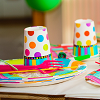Sitting Round the Party Tables

Age 5 to 11 Challenge Level:

Sweets are given out to party-goers in a particular way. Investigate the total number of sweets received by people sitting in different positions.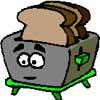Broken Toaster

Age 7 to 11 Short Challenge Level:

Only one side of a two-slice toaster is working. What is the quickest way to toast both sides of three slices of bread?Sums and Differences 1

Age 7 to 11 Challenge Level:

This challenge focuses on finding the sum and difference of pairs of two-digit numbers.Build it up More

Age 7 to 11 Challenge Level:

This task follows on from Build it Up and takes the ideas into three dimensions!Button-up Some More

Age 7 to 11 Challenge Level:

How many ways can you find to do up all four buttons on my coat? How about if I had five buttons? Six ...?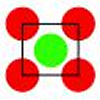Centred Squares

Age 7 to 11 Challenge Level:

This challenge, written for the Young Mathematicians' Award, invites you to explore 'centred squares'.Triangle Pin-down

Age 7 to 11 Challenge Level:

Use the interactivity to investigate what kinds of triangles can be drawn on peg boards with different numbers of pegs.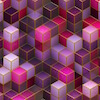Bundles of Cubes

Age 7 to 11 Challenge Level:

Watch this animation. What do you notice? What happens when you try more or fewer cubes in a bundle?Dice Stairs

Age 7 to 11 Challenge Level:

Can you make dice stairs using the rules stated? How do you know you have all the possible stairs?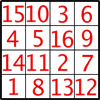Magic Constants

Age 7 to 11 Challenge Level:

In a Magic Square all the rows, columns and diagonals add to the 'Magic Constant'. How would you change the magic constant of this square?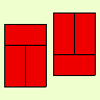Fault-free Rectangles

Age 7 to 11 Challenge Level:

Find out what a "fault-free" rectangle is and try to make some of your own.Cut it Out

Age 7 to 11 Challenge Level:

Can you dissect an equilateral triangle into 6 smaller ones? What number of smaller equilateral triangles is it NOT possible to dissect a larger equilateral triangle into?Calendar Calculations

Age 7 to 11 Challenge Level:

Try adding together the dates of all the days in one week. Now multiply the first date by 7 and add 21. Can you explain what happens?Round the Three Dice

Age 7 to 11 Challenge Level:

What happens when you round these three-digit numbers to the nearest 100?Simple Train Journeys

Age 5 to 11 Challenge Level:

How many different journeys could you make if you were going to visit four stations in this network? How about if there were five stations? Can you predict the number of journeys for seven stations?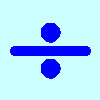Division Rules

Age 7 to 11 Challenge Level:

This challenge encourages you to explore dividing a three-digit number by a single-digit number.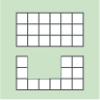Area and Perimeter

Age 7 to 11 Challenge Level:

What can you say about these shapes? This problem challenges you to create shapes with different areas and perimeters.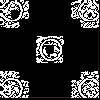Doplication

Age 7 to 11 Challenge Level:

We can arrange dots in a similar way to the 5 on a dice and they usually sit quite well into a rectangular shape. How many altogether in this 3 by 5? What happens for other sizes?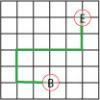Journeys in Numberland

Age 7 to 11 Challenge Level:

Tom and Ben visited Numberland. Use the maps to work out the number of points each of their routes scores.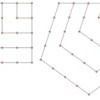Polygonals

Age 7 to 11 Challenge Level:

Polygonal numbers are those that are arranged in shapes as they enlarge. Explore the polygonal numbers drawn here.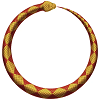Snake Coils

Age 7 to 11 Challenge Level:

This challenge asks you to imagine a snake coiling on itself.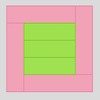Cuisenaire Rods

Age 7 to 11 Challenge Level:

These squares have been made from Cuisenaire rods. Can you describe the pattern? What would the next square look like?Age 5 to 11 Challenge Level:

Try out this number trick. What happens with different starting numbers? What do you notice?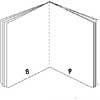Lost Books

Age 7 to 11 Challenge Level:

While we were sorting some papers we found 3 strange sheets which seemed to come from small books but there were page numbers at the foot of each page. Did the pages come from the same book?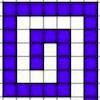Spirals, Spirals

Age 7 to 11 Challenge Level:

Here are two kinds of spirals for you to explore. What do you notice?Number Differences

Age 7 to 11 Challenge Level:

Place the numbers from 1 to 9 in the squares below so that the difference between joined squares is odd. How many different ways can you do this?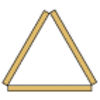Sticky Triangles

Age 7 to 11 Challenge Level:

Can you continue this pattern of triangles and begin to predict how many sticks are used for each new "layer"?Round the Dice Decimals 2

Age 7 to 11 Challenge Level:

What happens when you round these numbers to the nearest whole number?Always, Sometimes or Never?

Age 5 to 11 Challenge Level:

Are these statements relating to odd and even numbers always true, sometimes true or never true?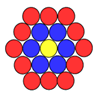Counting Counters

Age 7 to 11 Challenge Level:

Take a counter and surround it by a ring of other counters that MUST touch two others. How many are needed?Oddly

Age 7 to 11 Challenge Level:

Find the sum of all three-digit numbers each of whose digits is odd.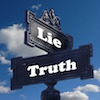Truth or Lie

Age 7 to 11 Challenge Level:

Take a look at the video of this trick. Can you perform it yourself? Why is this maths and not magic?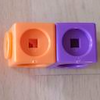Break it Up!

Age 5 to 11 Challenge Level:

In how many different ways can you break up a stick of 7 interlocking cubes? Now try with a stick of 8 cubes and a stick of 6 cubes.Round and Round the Circle

Age 7 to 11 Challenge Level:

What happens if you join every second point on this circle? How about every third point? Try with different steps and see if you can predict what will happen.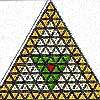The Great Tiling Count

Age 7 to 11 Challenge Level:

Compare the numbers of particular tiles in one or all of these three designs, inspired by the floor tiles of a church in Cambridge.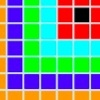Walking the Squares

Age 7 to 11 Challenge Level:

Find a route from the outside to the inside of this square, stepping on as many tiles as possible.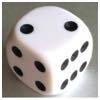Roll over the Dice

Age 7 to 11 Challenge Level:

Watch this video to see how to roll the dice. Now it's your turn! What do you notice about the dice numbers you have recorded?Window Frames

Age 5 to 14 Challenge Level:

This task encourages you to investigate the number of edging pieces and panes in different sized windows.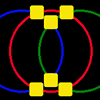Magic Circles

Age 7 to 11 Challenge Level:

Put the numbers 1, 2, 3, 4, 5, 6 into the squares so that the numbers on each circle add up to the same amount. Can you find the rule for giving another set of six numbers?Dotty Circle

Age 7 to 11 Challenge Level:

Watch this film carefully. Can you find a general rule for explaining when the dot will be this same distance from the horizontal axis?Round the Dice Decimals 1

Age 7 to 11 Challenge Level:

Use two dice to generate two numbers with one decimal place. What happens when you round these numbers to the nearest whole number?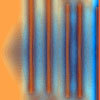Move a Match

Age 7 to 11 Challenge Level:

How can you arrange these 10 matches in four piles so that when you move one match from three of the piles into the fourth, you end up with the same arrangement?Have You Got It?

Age 11 to 14 Challenge Level:

Can you explain the strategy for winning this game with any target?Crossings

Age 7 to 11 Challenge Level:

In this problem we are looking at sets of parallel sticks that cross each other. What is the least number of crossings you can make? And the greatest?Domino Numbers

Age 7 to 11 Challenge Level:

Can you see why 2 by 2 could be 5? Can you predict what 2 by 10 will be?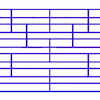Tumbling Down

Age 7 to 11 Challenge Level:

Watch this animation. What do you see? Can you explain why this happens?Shear Magic

Age 11 to 14 Challenge Level:

What are the areas of these triangles? What do you notice? Can you generalise to other "families" of triangles?